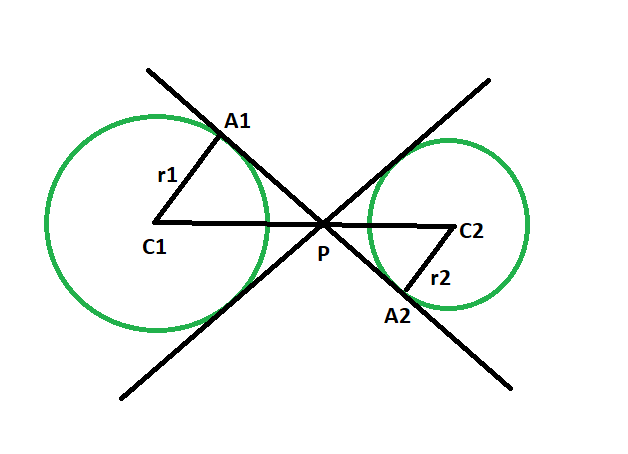GFG App
Open AppBrowser
Continue

# Ratio of the distance between the centers of the circles and the point of intersection of two transverse common tangents to the circles

Given two circles, of given radii, such that the circles don’t touch each other. The task is to find the ratio of the distance between the centres of the circles and the point of intersection of two transverse common tangents to the circles.
Examples:

```Input :r1 = 4, r2 = 8
Output :1:2

Input :r1 = 5, r2 = 13
Output :5:13```Approach

• Let the radii of the circles be r1 & r2 and C1 & C2 respectively.
• Let P be the point of intersection of two transverse common tangents to the circles, and A1 & A2 be the point of contact of the tangents with the circles.
• In triangle PC1A1 & triangle PC2A2
angle C1A1P = angle C2A2P = 90 deg { line joining the centre of the circle to the point of contact makes an angle of 90 degree with the tangent },
also, angle A1PC1 = angle A2PC2{vertically opposite angles are always equal}
so, angle A1C1P = angle A2C2P
as angles are same, triangles PC1A1 & PC2A2 are similar.
• So, due to similarity of the triangles,
C1P/C2P = C1A1/C2A2 = r1/r2## C++

 `// C++ program to find the ratio` `// of the distance between the centres of the circles` `// and the point of intersection` `// of two transverse common tangents` `// to the circles which do not touch each other`   `#include ` `using` `namespace` `std;`   `int` `GCD(``int` `a, ``int` `b)` `{` `    ``return` `(b != 0 ? GCD(b, a % b) : a);` `}`   `// Function to find the ratio` `void` `ratiotang(``int` `r1, ``int` `r2)` `{` `    ``cout << ``"The ratio is "` `         ``<< r1 / GCD(r1, r2)` `         ``<< ``":"` `         ``<< r2 / GCD(r1, r2)` `         ``<< endl;` `}`   `// Driver code` `int` `main()` `{` `    ``int` `r1 = 4, r2 = 8;` `    ``ratiotang(r1, r2);` `    ``return` `0;` `}`

## Java

 `// Java program to find the ratio` `// of the distance between the centres of the circles` `// and the point of intersection` `// of two transverse common tangents` `// to the circles which do not touch each other`   `import` `java.io.*;`   `class` `GFG{`   `    ``static` `int` `GCD(``int` `a, ``int` `b)` `    ``{` `        ``return` `(b != ``0` `? GCD(b, a % b) : a);` `    ``}`   `    ``// Function to find the ratio` `    ``static` `void` `ratiotang(``int` `r1, ``int` `r2)` `    ``{` `        ``System.out.println(``"The ratio is "` `            ``+ r1 / GCD(r1, r2)` `            ``+ ``":"` `            ``+ r2 / GCD(r1, r2));` `    ``}`   `    ``// Driver code` `    ``public` `static` `void` `main (String[] args)` `    ``{` `        ``int` `r1 = ``4``, r2 = ``8``;` `        ``ratiotang(r1, r2);` `    ``}` `}`   `// This code is contributed by NamrataSrivastava1`

## Python

 `# Python3 program to find the ratio` `# of the distance between the centres of the circles` `# and the point of intersection` `# of two transverse common tangents` `# to the circles which do not touch each other`   `def` `GCD(a, b):` `    ``if``(b!``=``0``):` `        ``return` `GCD(b, a``%``b);` `    ``else``:` `        ``return` `a;`   `# Function to find the ratio` `def` `ratiotang(r1, r2):`   `    ``print``(``"The ratio is"``, r1 ``/``/` `GCD(r1, r2), ` `                     ``":"``, r2 ``/``/` `GCD(r1, r2));`   `# Driver code` `r1 ``=` `4``; r2 ``=` `8``;` `ratiotang(r1, r2);`   `# This code is contributed by Code_Mech`

## C#

 `// C# program to find the ratio` `// of the distance between the centres of the circles` `// and the point of intersection` `// of two transverse common tangents` `// to the circles which do not touch each other` `using` `System;`   `class` `GFG` `{`   `    ``static` `int` `GCD(``int` `a, ``int` `b)` `    ``{` `        ``return` `(b != 0 ? GCD(b, a % b) : a);` `    ``}`   `    ``// Function to find the ratio` `    ``static` `void` `ratiotang(``int` `r1, ``int` `r2)` `    ``{` `        ``Console.WriteLine(``"The ratio is "` `            ``+ r1 / GCD(r1, r2)` `            ``+ ``":"` `            ``+ r2 / GCD(r1, r2));` `    ``}`   `    ``// Driver code` `    ``static` `public` `void` `Main ()` `    ``{` `        `  `        ``int` `r1 = 4, r2 = 8;` `        ``ratiotang(r1, r2);` `    ``}` `}`   `// This code is contributed by Tushil.`

## PHP

 ``

## Javascript

 ``

Output:

`The ratio is 1:2`

Time Complexity: O(log(min(a, b)))

Auxiliary Space: O(log(min(a, b)))

My Personal Notes arrow_drop_up